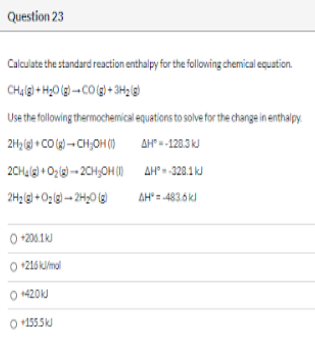# Calculate the standard reaction enthalpy for the following chemical equation. CH4(g) + H2O(g) → CO(g) + 3H2(g) Use the following thermochemical equations to solve for the change in enthalpy. 2H2(g) + CO(g) → CH3OH(l) ΔH° = -128.3 kJ 2CH4(g) + O2(g) → 2CH3OH(l) ΔH° = -328.1 kJ 2H2(g) + O2(g) →2H2O(g) ΔH° = -483.6 kJ a) +206.1 kJ b) +216 kJ/mol c) +42.0 kJ d) +155.5 kJ